Next: 3.3.5 Boundaries Up: 3.3.4 Semiconductor-Metal Interfaces: The Previous: 3.3.4.2 The Ohmic Contact:

### 3.3.4.3 Schottky Contact

For the gate contact in the HEMT two models are commonly used to model the Schottky contact. The first model for the contact interface condition for the potential is: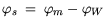(3.111)

where: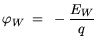(3.112)

Thus, the potential at the boundary in the semiconductor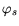is set to the difference of the quasi-Fermi level potential in the metal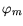, which is equal to the potential specified at the contact, and the potential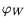. The latter represents the potential corresponding to the work function difference energy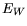of metal and semiconductor. The applied current equations are: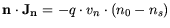(3.113)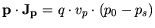(3.114)

The currents are proportional to the so-called recombination velocities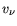and the difference of the carrier concentration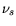and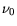. The carrier concentrations at the boundary read: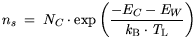(3.115)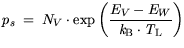(3.116)

while the equilibrium concentrations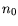and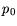can be expressed as: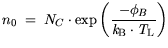(3.117)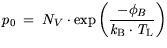(3.118)

For the Schottky barrier height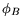typical values range between 0.61 eV and 0.8 eV. For the hydrodynamic case the carrier temperatures are fixed, thus thermal equilibrium is assumed similarly to the Ohmic contact. The thermal boundary conditions applied similar to the Ohmic contact, i.e. either by an thermal resistance or a isothermal boundary condition. The model presented so far can also be called a thermionic emission model: The recombination velocityin (3.113) is then to be called a thermionic emission velocity. Inserting (3.115) and (3.117) into (3.113) we obtain: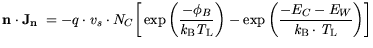(3.119)

As stated by Schroeder in , (3.119) can thus be written as: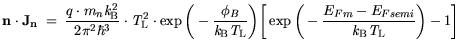(3.120)

using=-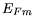which is already named the Schottky barrier height. Furthermore we use: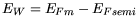(3.121)

which says that the work function difference is equal to the difference of the Fermi levels of the semiconductor and the metal. The velocity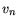is rewritten as (see (3.92)):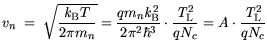(3.122)

where A is the Richardson constant typical for thermionic emission processes. Thus, (3.122) represents a typical thermionic emission equation, which is determined by the Schottky barrier heightand the difference of the Fermi levels. Neglecting the tunneling in (3.91) the similarities are obvious. Typically the Fermi level of the semiconductor is chosen as the reference energy. A second model for the metal semiconductor interface including a tunneling current component has been tested [130,174]. It is based of the equivalence of a tunneling current and a generation process. It was found that such a non-local model is physically sound and useful on the first glance, but its usefulness depends critically on the actual two-dimensional implementation of the integration path for the tunneling probability in general purpose simulators, as stated in Section 3.6.Next: 3.3.5 Boundaries Up: 3.3.4 Semiconductor-Metal Interfaces: The Previous: 3.3.4.2 The Ohmic Contact:
Quay
2001-12-21